# ADDITION OF 2-DIGIT NUMBERS WITH REGROUPING

HELLO CHILDREN, WELCOME TO A CLASS

Here we will learn adding 2-digit numbers with regrouping and start working with easy numbers to get acquainted with the addition of two numbers.

How to add two two-digit numbers with carrying?

Let us follow the steps and start learning adding 2-digit numbers with regrouping with the help of these examples.

1. 45 + 37

(i) First arrange the numbers vertically so that the tens’ place digits and ones’ place digits are lined up which means in simple one number should be written above the other number. Draw a line under the bottom number.

(ii) Add the ones place digits. Add (5 + 7 = 12). Place 2 in the one’s column and place 1 above the tens column as shown.

(iii) Then add the tens’ place digits. Add (4 + 3 + 1 = 8) and place 8 in the tens as column as shown

(iv) The answer of the sum 45 + 37 is 82..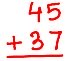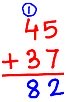1. 58 + 39

Method II: 58 + 39

• 8 ones + 9 ones = 17 units (Regroup 17 units)
• 17 units = 10 units + 7 units = 1 ten + 7 ones
• Place 7 in the ones answer column and place 1 in the tens carry-up column.
• 5 tens +3 tens + 1 tens = 9 tens
• Place 9 in the tens answer column.
• So, adding 58 + 39 = 97.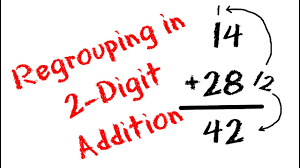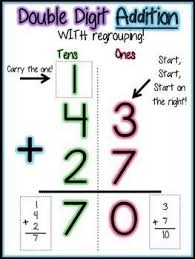ACTIVITY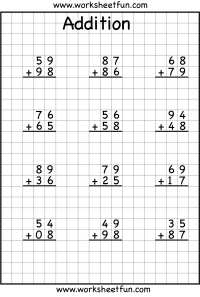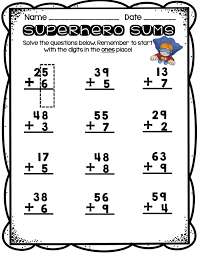How Can We Make ClassNotesNG Better? - CLICK to Tell Us Now!